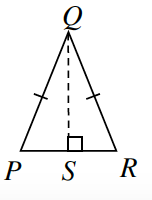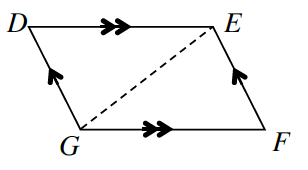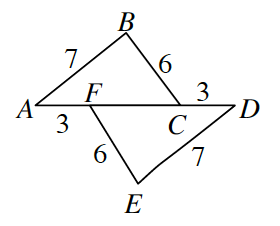### Home > GB8I > Chapter 8 Unit 9 > Lesson INT1: 8.1.1 > Problem8-11

8-11.

For each pair of triangles below, determine if the triangles are congruent. If the triangles are congruent, complete the congruence statement and state the congruence property. Otherwise, explain why you cannot conclude that the triangles are congruent.

1. $ΔSQP ≅ Δ\text{____}$$ΔSQR$; $HL≅$

($\overline{QS}≅SQ$, Reflexive Property)

1. $ΔEDG ≅ Δ\text{____}$$ΔGFE$; $\text{ASA}≅$

($\overline{GE}≅\overline{EG}$ Reflexive Property)

1. $ΔABC ≅ Δ\text{____}$$ΔDEF$; $\text{SSS}≅$